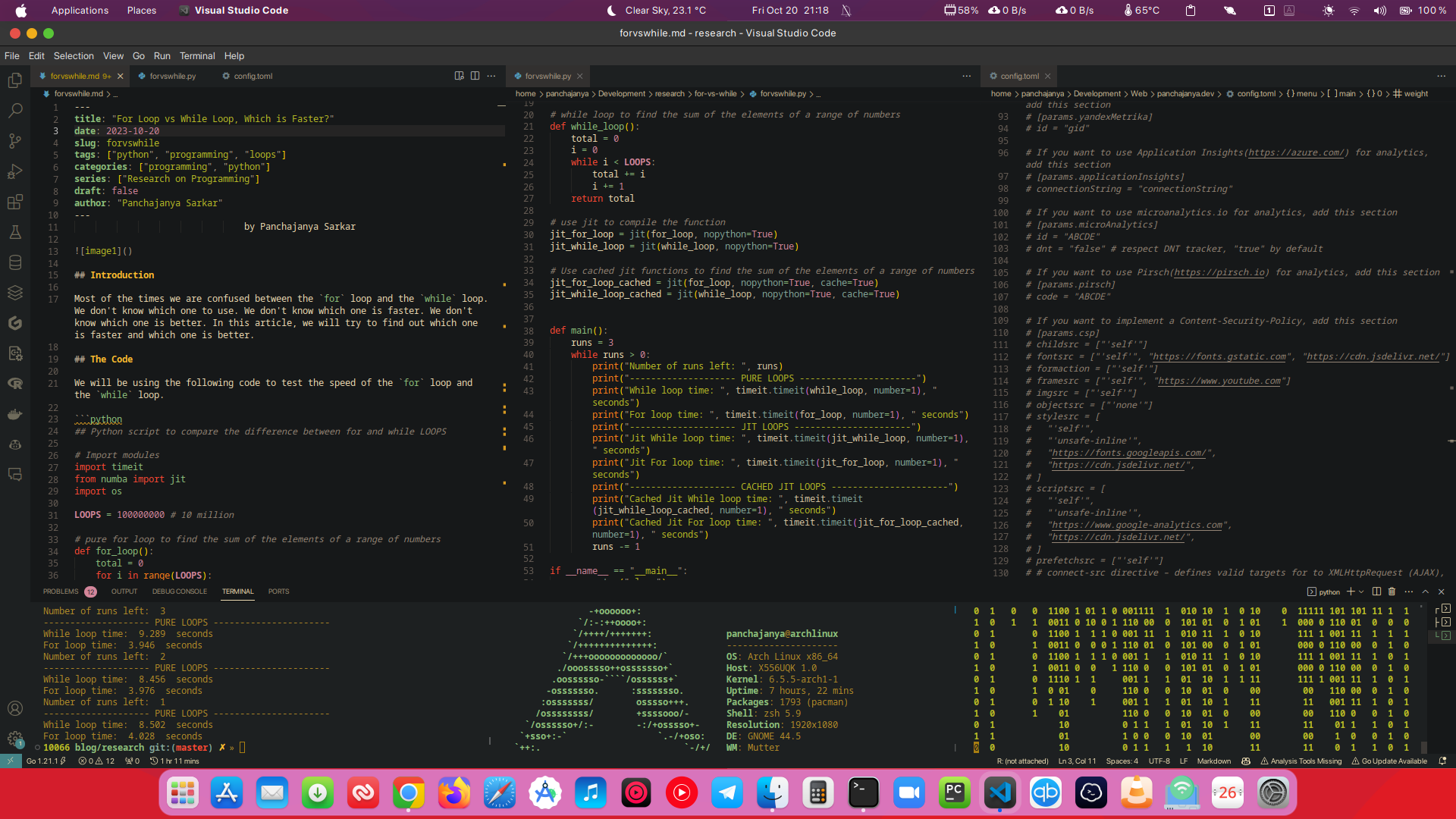# For Loop vs While Loop, Which is Faster?

by Panchajanya SarkarMost of the times we are confused between the `for` loop and the `while` loop. We don’t know which one to use. We don’t know which one is faster. We don’t know which one is better. In this article, we will try to find out which one is faster and which one is better.

We will be using the following code to test the speed of the `for` loop and the `while` loop.

Note
Run this code on Replit
``````## Python script to compare the difference between for and while LOOPS

# Import modules
import os
import timeit

LOOPS = 100000000 # 10 million

# pure for loop to find the sum of the elements of a range of numbers
def for_loop():
total = 0
for i in range(LOOPS):
total += i

# while loop to find the sum of the elements of a range of numbers
def while_loop():
total = 0
i = 0
while i < LOOPS:
total += i
i += 1

def main():
runs = 3
while runs > 0:
print("Number of runs left: ", runs)
print("-------------------- PURE LOOPS ----------------------")
print("While loop time: ", timeit.timeit(while_loop, number=1), " seconds")
print("For loop time: ", timeit.timeit(for_loop, number=1), " seconds")
runs -= 1

if __name__ == "__main__":
# clear the screen
os.system("clear")
main()
``````

Let’s understand the codes one by one. One function at a time!

### The `for` loop Link to heading

``````LOOPS = 100000000 # 10 million
# pure for loop to find the sum of the elements of a range of numbers
def for_loop():
total = 0
for i in range(LOOPS):
total += i
``````

This `for` loop is a pretty basic `for` loop which basically iterates through the range of 10 million digits, starting from 0. The variable `total` inside the loop stores the sum of each iteration and finally returns the sum of all the eleements in the given range. Here, `i` is used to iterate throught the range of digits. The loop runs until the value of `i` is less than 10 million.

### The `while` loop Link to heading

``````LOOPS = 100000000 # 10 million
# while loop to find the sum of the elements of a range of numbers
def while_loop():
total = 0
i = 0
while i < LOOPS:
total += i
i += 1
``````

This while loop also calculates the sum of the elements of a range of numbers. The range of numbers is from 0 to 10 million. The `while` loop is used to iterate over the range of numbers and add them to the variable `total`. The `total` variable is then returned. Check here, i is used which is the counter variable. The counter variable is incremented by 1 in each iteration. The loop will run until the counter variable is less than 10 million.

We ran the code 3 times and timed the execution of the code on my Linux Machine. The results are as follows:

Run 1

``````Number of runs left:  3
-------------------- PURE LOOPS ----------------------
While loop time:  9.289  seconds
For loop time:  3.946  seconds
``````

Run 2

``````Number of runs left:  2
-------------------- PURE LOOPS ----------------------
While loop time:  8.456  seconds
For loop time:  3.976  seconds
``````

Run 3

``````Number of runs left:  1
-------------------- PURE LOOPS ----------------------
While loop time:  8.502  seconds
For loop time:  4.028  seconds
``````

The results are pretty clear. The `for` loop is faster than `while` loop. Can you have the slightest guess why? If you can’t, then let me explain it to you.
Well, let’s explain this in layman’s terms, the `for` loop in Python is faster than `while` loop because of the way the interpreter optimizes the operation. It’s designed to iterate over items from collections, iterators which allows the language to process these using efficient C loops under the hood, rather havng to manage loop counts or condiotions at the Python level. This leads to signoficant speed and performance gains.
So, here we have seen that the `for` loop is much faster than the `while` loop. So, we should use the `for` loop whenever possible. But, there are some cases where we have to use the `while` loop. For example, when we don’t know the number of iterations, we have to use the `while` loop. But, if we know the number of iterations, we should use the `for` loop.